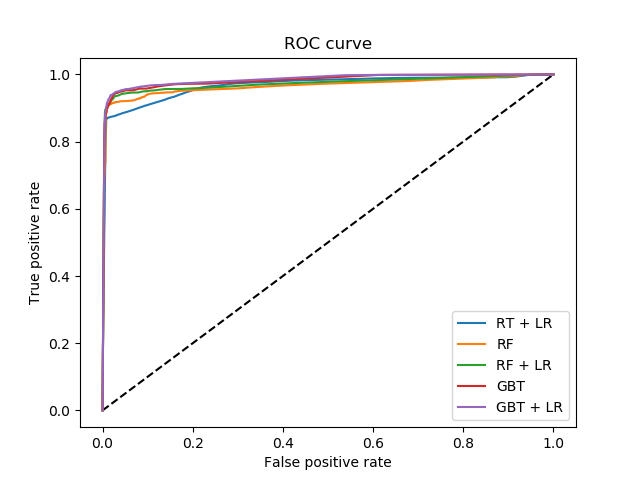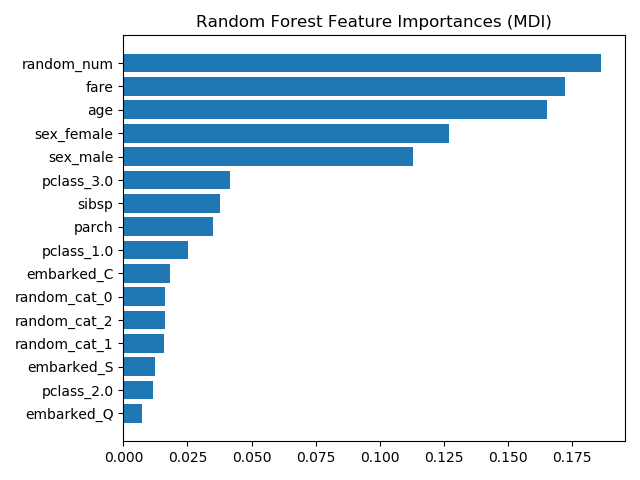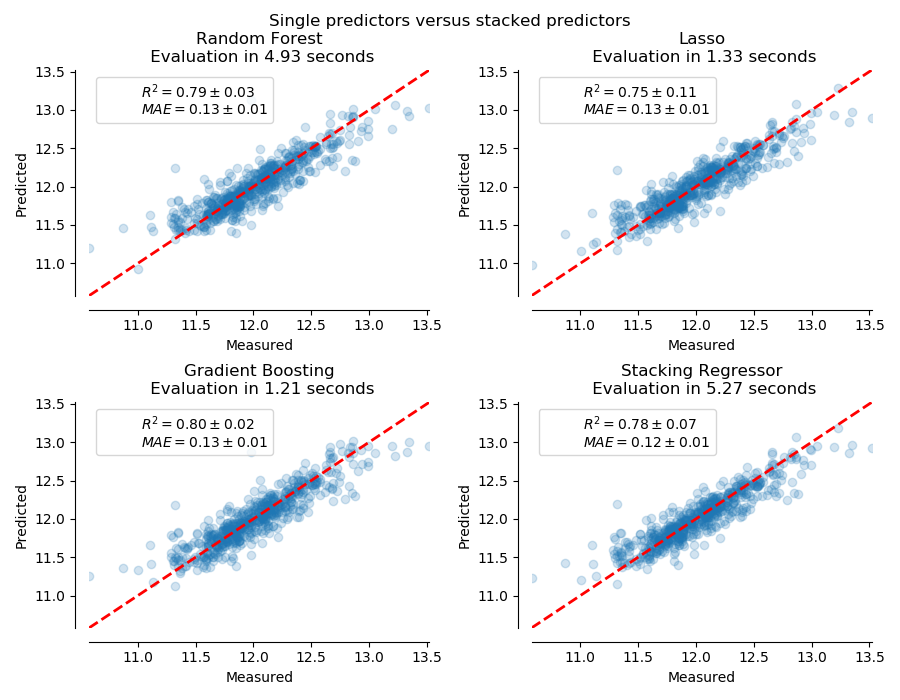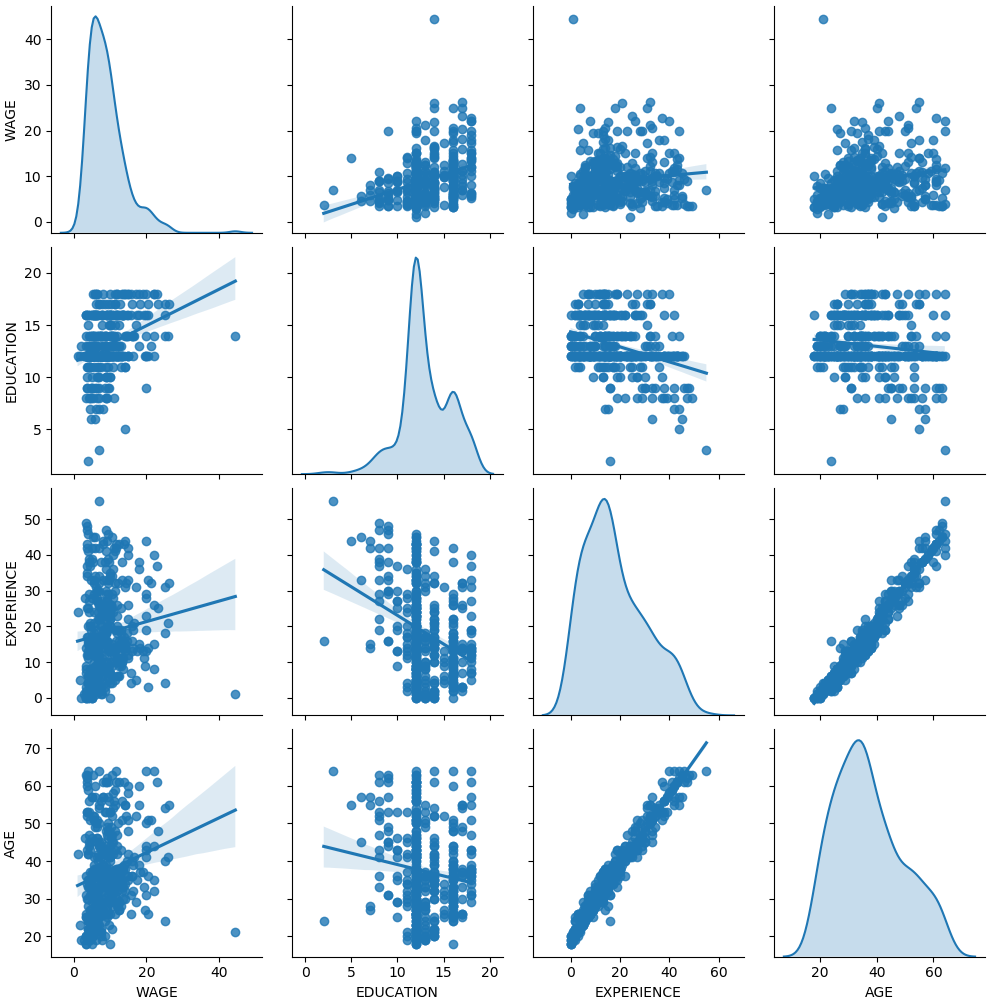# sklearn.preprocessing.OneHotEncoder¶

class sklearn.preprocessing.OneHotEncoder(*, categories='auto', drop=None, sparse=True, dtype=<class 'numpy.float64'>, handle_unknown='error')

categories ‘auto’ or a list of array-like, default=’auto’

‘auto’：从训练数据中自动确定类别。
list：category [i]保存第i列中期望的类别。传递的类别不应在单个功能中混合使用字符串和数字值，并且应该对数字值进行排序。

0.20版中的新功能。
drop {‘first’, ‘if_binary’} or a array-like of shape (n_features,), default=None

None：保留所有功能（默认）。
‘first’：在每个功能中删除第一类。如果仅存在一个类别，则该功能将被完全删除。
‘if_binary’：将具有两个类别的每个特征的第一个类别删除。具有1个或2个以上类别的要素保持不变。
array：drop [i]是要素X [:, i]中应删除的类别。
sparse bool, default=True

dtype number type, default=np.float

handle_unknown {‘error’, ‘ignore’}, default=’error’

categories_ list of arrays

drop_idx_ array of shape (n_features,)
drop_idx_ [i]是要为每个功能删除的类别的category_ [i]中的索引。

sklearn.preprocessing.OrdinalEncoder

sklearn.feature_extraction.DictVectorizer

sklearn.feature_extraction.FeatureHasher

sklearn.preprocessing.LabelBinarizer

sklearn.preprocessing.MultiLabelBinarizer

>>> from sklearn.preprocessing import OneHotEncoder

>>> enc = OneHotEncoder(handle_unknown='ignore')>>> X = [['Male', 1], ['Female', 3], ['Female', 2]]>>> enc.fit(X)OneHotEncoder(handle_unknown='ignore')>>> enc.categories_[array(['Female', 'Male'], dtype=object), array([1, 2, 3], dtype=object)]>>> enc.transform([['Female', 1], ['Male', 4]]).toarray()array([[1., 0., 1., 0., 0.],       [0., 1., 0., 0., 0.]])>>> enc.inverse_transform([[0, 1, 1, 0, 0], [0, 0, 0, 1, 0]])array([['Male', 1],       [None, 2]], dtype=object)>>> enc.get_feature_names(['gender', 'group'])array(['gender_Female', 'gender_Male', 'group_1', 'group_2', 'group_3'],  dtype=object)

>>> drop_enc = OneHotEncoder(drop='first').fit(X)>>> drop_enc.categories_[array(['Female', 'Male'], dtype=object), array([1, 2, 3], dtype=object)]>>> drop_enc.transform([['Female', 1], ['Male', 2]]).toarray()array([[0., 0., 0.],       [1., 1., 0.]])

>>> drop_binary_enc = OneHotEncoder(drop='if_binary').fit(X)>>> drop_binary_enc.transform([['Female', 1], ['Male', 2]]).toarray()array([[0., 1., 0., 0.],       [1., 0., 1., 0.]])

fit(X[, y]) 使OneHotEncoder适应X。
fit_transform(X[, y]) 使OneHotEncoder拟合X，然后变换X。
get_feature_names([input_features]) 返回输出要素的要素名称。
get_params([deep]) 获取此估计量的参数。
inverse_transform(X) 将数据转换回原始表示形式。
set_params(**params) 设置此估算器的参数。
transform(X) 使用one-hot编码转换X。
__init__(*, categories='auto', drop=None, sparse=True, dtype=<class 'numpy.float64'>, handle_unknown='error')

[源码]

fit(X, y=None)

[源码]

X array-like, shape [n_samples, n_features]

y None

self -
fit_transform(X, y=None)

[源码]

X array-like, shape [n_samples, n_features]

y None

X_out sparse matrix if sparse=True else a 2-d array

get_feature_names(input_features=None)

[源码]

input_features list of str of shape (n_features,)

output_feature_names ndarray of shape (n_output_features,)

get_params(deep=True)

[源码]

deep bool, default=True

params mapping of string to any

inverse_transform(X)

[源码]

X array-like or sparse matrix, shape [n_samples, n_encoded_features]

X_tr array-like, shape [n_samples, n_features]

set_params(**params)

[源码]

**params dict

self object

transform(X)

[源码]

X array-like, shape [n_samples, n_features]

X_out sparse matrix if sparse=True else a 2-d array

## sklearn.preprocessing.OneHotEncoder使用示例¶